# matlab简单图像处理

## MATLAB一些简单的图像处理程序

2016-10-10 18:18:48 yangkunpengD 阅读数 9229

1

```I=imread('pout.tif');
figure,imshow(I);
[m,n]=size(I);
Inew=zeros(floor(m/2),n);
for i=1:m
for j=1:n
Inew(i,j)=I(2*i-1,j);
end
end
figure,imshow(uint8(Inew))```

2

```clc
clear
x=0:255;
y1=x;
figure,
plot(x,y1,'r')
hold on
y2=255:-1:0;
plot(x,y2,'b')
ylog=255*(log(x+1)/log(256));
plot(x,ylog,'g+')
yexp=255*x.^3/255^3;
plot(x,yexp,'y+')
c=255/255^3;
ynthroot=nthroot((x/c),3);
plot(x,ynthroot,'yo')```
3

```I=imread('moon.tif');
I=double(I);
[m,n]=size(I);
for i=1:m
for j=1:n
if I(i,j)<100
Inew(i,j)=3*I(i,j);
elseif I(i,j)>200
Inew(i,j)=0.8*I(i,j);
else
Inew(i,j)=I(i,j);
end
end
end
figure,imshow(Inew,[])```
4

```clear
clc
I=im2double(I);
[m,n]=size(I);
for i=1:m
for j=1:n
Inew(i,j)=I(i,j)^0.2;
end
end
figure,imshow(I,[])
figure,imshow(Inew,[])```
5

```clear
clc
I=im2double(I);
[m,n]=size(I);
%循环实现
tic
for i=1:m
for j=1:n
Inew(i,j)=255*log(I(i,j)+1)/log(256);
end
end
toc
%查找表
tic
x=0:255;
c=255/log(256);
y=c*log(x+1);
I_log=uint8(y(x+1));
figure,plot(x,y)
toc
figure,imshow(I_log,[])
figure,imshow(I,[])
figure,imshow(Inew,[])```

6

```I=imread('pout.tif');
figure,imshow(I)
[m,n]=size(I);
h=zeros(1,256);
for k=0:255
for i=1:m
for j=1:n
if(I(i,j)==k)
h(k+1)=h(k+1)+1;
end
end
end
end
figure,bar(h)```

```I=imread('pout.tif');
figure,imshow(I)
[m,n]=size(I);
h=zeros(1,256);
for i=1:m
for j=1:n
h(I(i,j)+1)=h(I(i,j)+1)+1;
end
end
figure,bar(h)```

```I=imread('pout.tif');
figure,imshow(I)
[m,n]=size(I);
h=zeros(1,256);
for i=1:m
for j=1:n
h(I(i,j)+1)=h(I(i,j)+1)+1;
end
end
figure,bar(h)
p=h/(m*n);
sum=0;
for i=1:256
sum=sum+p(i);
y(i)=round(sum*255);
end
for i=1:m
for j=1:n
Inew(i,j)=y(I(i,j)+1);
end
end

figure,imshow(unit8(Inew))```

7 邻域处理

```I=imread('pout.tif');
I=double(I);
%卷积模板的不同处理效果不同
%M=ones(3,3)/9;将一幅图模糊处理； M=[0 -1 0;-1 5 -1;0 -1 0];将一幅图锐化处理，改变中心位置的值有不同效果
%M=[1 1 1;0 0 0;-1 -1 -1];指出原始图像中水平边缘存在位置的图像；M=[1 0 -1;1 0 -1;1 0 -1];指出原始图像中垂直边缘存在位置的图像
M=ones(3,3)/9;
[m,n]=size(I);
Inew=zeros(m,n);
%从2开始到m-1忽略边界
for i=2:m-1
for j=2:n-1
Inew(i,j)=I(i-1,j-1)*M(1,1)+I(i-1,j)*M(1,2)+...
I(i-1,j+1)*M(1,3)+I(i,j-1)*M(2,1)+I(i,j)*M(2,2)+...
I(i,j+1)*M(2,3)+I(i+1,j-1)*M(3,1)+I(i+1,j)*M(3,2)+...
I(i+1,j+1)*M(3,3);
end
end
figure,imshow(uint8(Inew))```

matlab简单图像处理 相关内容

## matlab数字图像处理实验

2018-01-08 18:41:26 aninstein 阅读数 5219

（二）matlab数字图像处理实验-图像的几何变换

matlab简单图像处理 相关内容

## MATLAB数字图像处理（一）图像的基本操作

2015-10-12 19:47:54 zxc024000 阅读数 89248

有人问，处理图片，用PS岂不是更好。两者各有优点，如果需要将1００００幅图片转换成灰度图像并保存呢？MATLAB只需要一段很短的程序运行几秒就可以完成这个工作。

# MATLAB中图像的基本操作

## 1、读取、显示图片

``````imread：

A是结构体名，用来存储读入的图像数据。filename是读取的文件名，文件名要用”括起来。fmt是读取文件的类型如：jpg、png等等，这个参数可以不输入，由MATLAB自动判断。

``````    imshow：
imshow(I)``````

I为读取后保存在MATLAB中的结构体名。

``````A=imread('1.jpg');％读取名为１．ｊｐｇ的图片
imshow(A)％显示图片``````## ２、将灰度图片变成负片

对图像进行操作，实际上是将图像看成许多个像素点，对每个像素点进行操作。在计算机系统中，灰度图片被看成是许多个由值在［０～２５５］之间的像素点组成的图像，２５５表示白色，０表示黑色，黑白之间存在２５６个灰度级。负片是指将原灰度图白色的地方变成黑色，黑色的地方变成白色。也就是将０变成２５５，２５５变成０。ＭＡＬＴＡＢ的ｉｍａｄｊｕｓｔ（）函数提供了该功能。其函数原型：

``````imadjust：

其中，Ｉ为原灰度图像，ｌｏｗ＿ｉｎ，ｈｉｇｈ＿ｉｎ为输入图像的低和高灰度级，设置为［０，１］表示从０～２５５的归一化，ｌｏｗ＿ｏｕｔ，ｈｉｇｈ＿ｏｕｔ为输出图像的低高灰度级。
若是想将图片转换为负片，那么将[low_in; high_in]设置为［０，１］，将[low_out; high_out]设置为［１，０］。即原来输入为０的地方变成１输出，输入为１的地方变成０输出。
实例：

``````　A=imread('1.jpg');
imshow(A)％显示原图
figure,imshow(A1)％显示负片``````## ３、彩色图片转换成灰度图片

我们在计算机中看到的大多数彩色图片是基于ＲＢＧ三通道的图片，红绿蓝三种颜色，每一种取值均为［０～２５５］。通过２５５＊２５５＊２５５，可以构成庞大的颜色群。而灰度图像只有２５６个灰度级。通过以下公式可以将ＲＧＢ转换成灰度
ＧＲＡＹ＝0.2989 R + 0.5870 * G + 0.1140 * B*
ＭＡＴＬＡＢ中提供的函数ｒｇｂ２ｇｒａｙ为我们提供了将彩色图片转换成灰度图片的功能。函数原型：

``I = rgb2gray(RGB)``

`````` A=imread('001.png');％原彩色图片
imshow(A)
A_gray=rgb2gray(A);％转换成灰度图片
figure,imshow(A_gray)``````matlab简单图像处理 相关内容

## MATLAB简单的图像处理

``````**%% **1、生成10*10的二位随机矩维****
clc;
clear all;
A=rand(10,10);%生成10*10的随机矩阵
A(3:5,5:7)=0;%3到5行,5到7列置位0;
B=A';%求A的转置
%裁剪
A(2,:)%提取A的第二行
A(:,2)%提取A的第二列
x````````````**%% 2、冒泡法实现将10个元素的排序**
clc;
clear all;
a=[3,2,4,1,6,5,9,7,8,0];
a
for j=9:-1:1
for i=1:j
if(a(i)>a(i+1))
t=a(i);a(i)=a(i+1);a(i+1)=t;
end
end
end
a````````````**%% 3、创建m文件，实现图像的读取，显示，保存以及信息观察;**
clc;
clear all;
figure:imshow(R);%显示图像
imwrite(R,'C:\Users\东\Desktop\autumn.tif');%信息保存````````````**%% 4、运行代码观察其中变量A与B的值和类型，写上注释，分析图一和图二不同，又为何图一和图三相同**
clc;
clear all;
B=double(A);%复制一份A
subplot(1,3,1),imshow(A);%在一个1行3列的图中定位第一个图来进行操作并显示图像
subplot(1,3,2),imshow(B);%在一个1行2列的图中定位第一个图来进行操作并显示图像
subplot(1,3,3);imshow(B,[0,255]);%显示B，并将B的值映射为0（纯黑）到255（纯白）之间的标准灰度值
%图一和图二不同B在复制A的同时没有调整灰度值，以纯白的形式显示出来
%图一和图三相同是因为B的值映射为0（纯黑）到255（纯白）之间的标准灰度值显示出来``````matlab简单图像处理 相关内容

## 使用matlab进行简单图像处理

2019-09-26 21:35:58 qq_36808245 阅读数 9871、原始图像

2、左右翻转的图像

3、上下翻转的图像

4、放大一倍的图像

5、显示其直方图

6、图像向右下方平移半个图像长宽的距离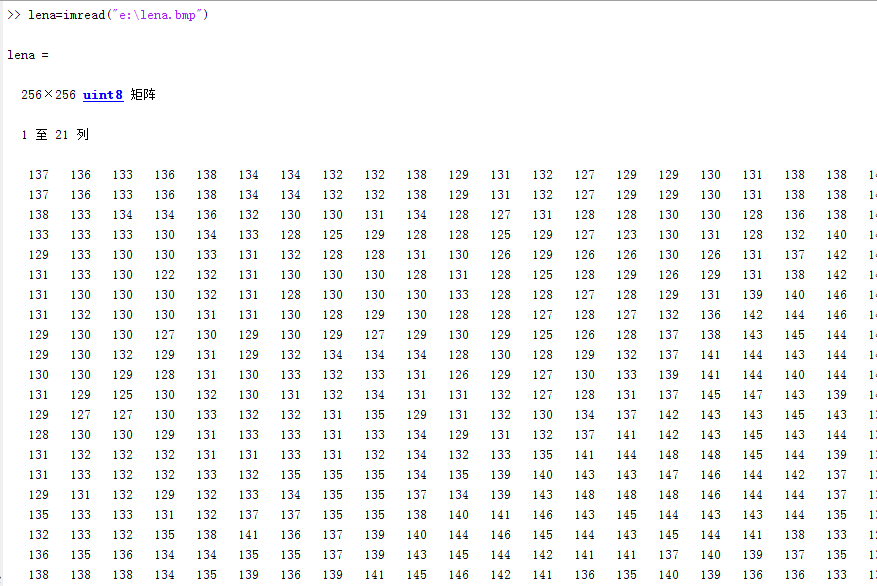### 读取图像并显示原始图像

imshow(lena);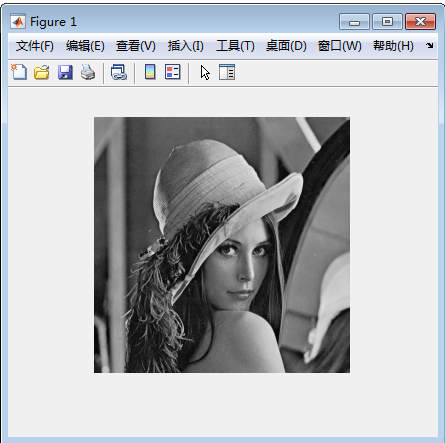### 左右翻转的图像

lena2=flip(lena,2);
imshow(lena2);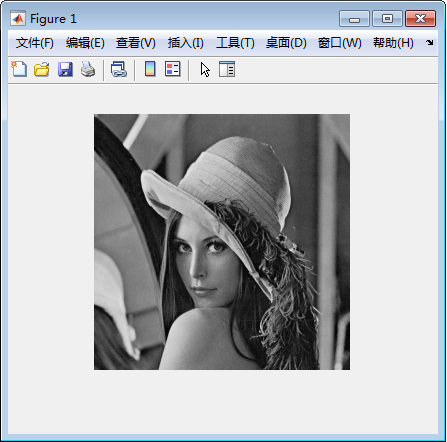### 上下翻转的图像

lena3=flip(lena);
imshow(lena3);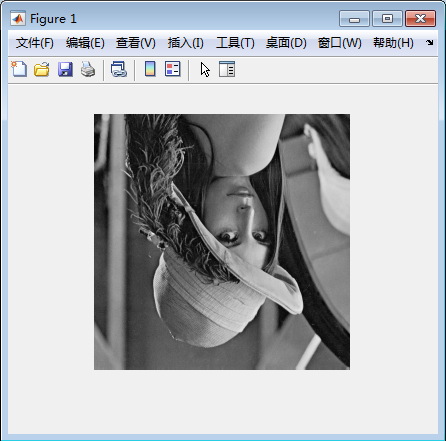### 放大一倍的图像

lena4=imresize(lena,2);
imshow(lena4);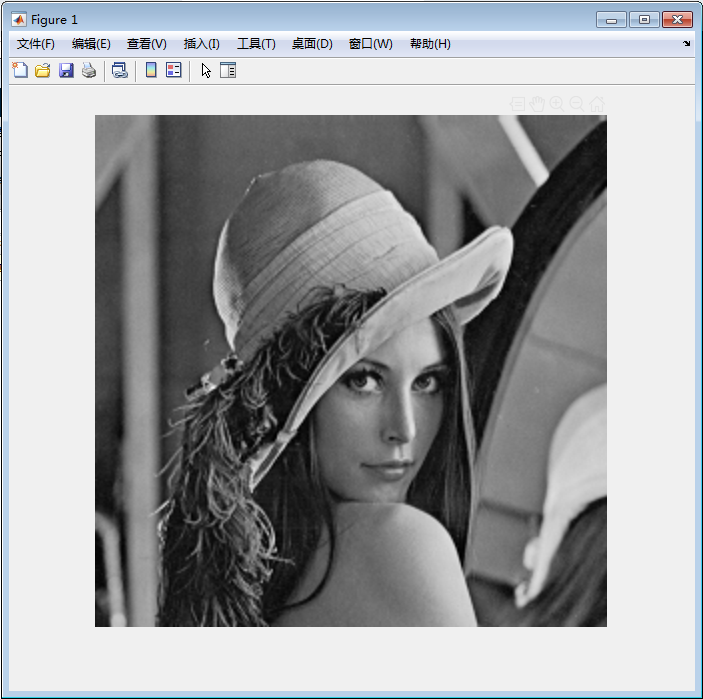### 显示其直方图

h=histogram(lena,255)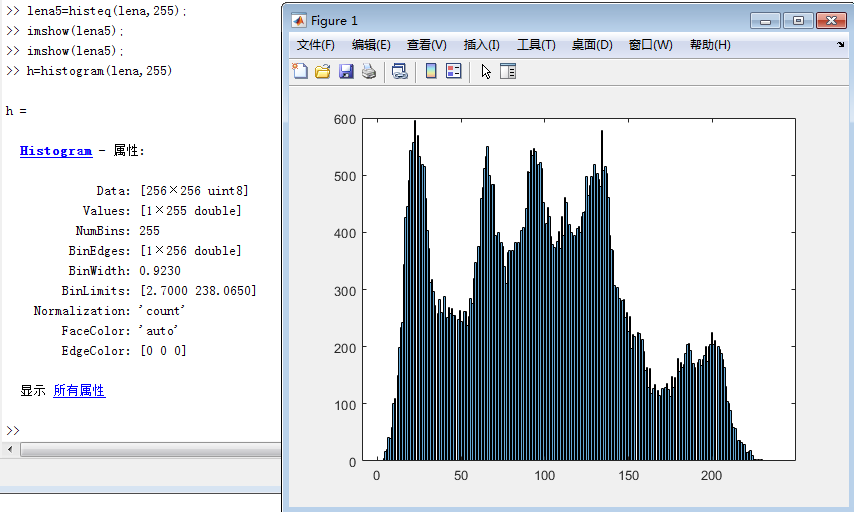imshow(lena5)显示的是一个比lena要更亮一点的图片（直方图均衡化）

###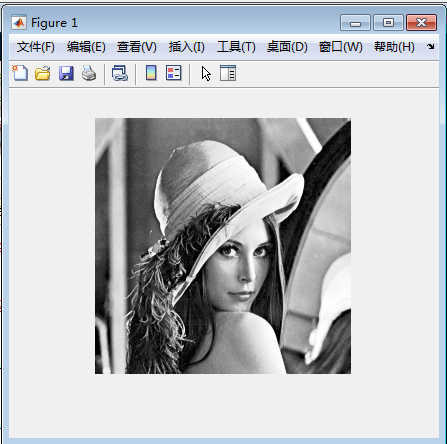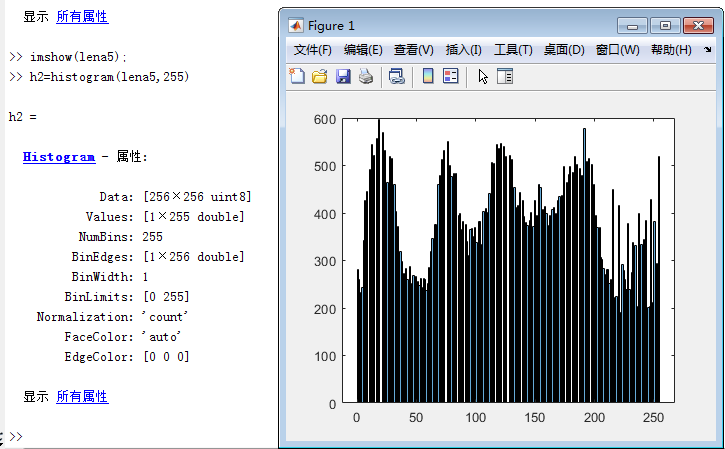图像向右下方平移半个图像长宽的距离

``````% lena=imread("e:\lena.bmp");
[R, C] = size(lena); % 获取图像大小
res = zeros(R, C); % 构造结果矩阵。每个像素点默认初始化为0（黑色）
delX = 128; % 平移量X 原始图像的一半直接256/2指定128了，
% delX = R/2; % 平移量X
% delY = C*0.5; % 平移量Y，这些写法都可以
delY = 128; % 平移量Y
tras = [1 0 delX; 0 1 delY; 0 0 1]; % 平移的变换矩阵

for i = 1 : R
for j = 1 : C
temp = [i; j; 1];
temp = tras * temp; % 矩阵乘法
x = temp(1, 1);
y = temp(2, 1);
% 变换后的位置判断是否越界
if (x <= R) & (y <= C) & (x >= 1) & (y >= 1)
res(x, y) = lena(i, j);
end
end
end;

imshow(uint8(res)); % 显示图像``````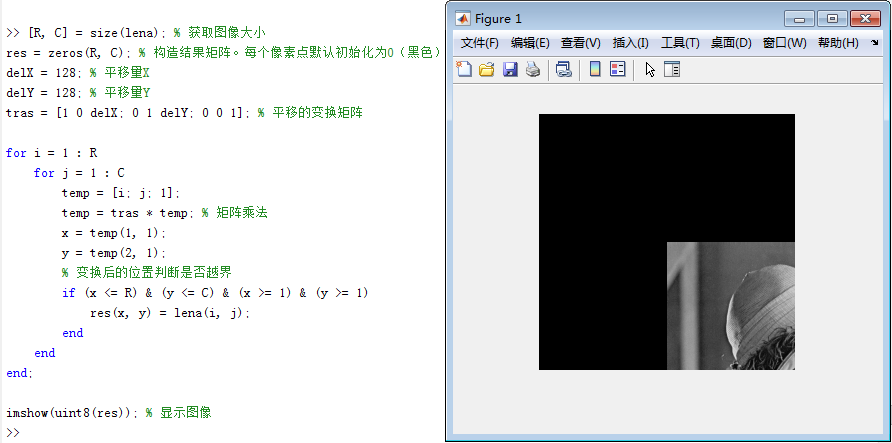``````se=translate(strel(1),[128 128]);%构造结构元素
res=imdilate(lena,se);%形态学膨胀
figure;%显示图片框，以下内容都会显示在里面
subplot(1,2,1),subimage(lena);
title('orginal image');
subplot(1,2,2),subimage(res);
title('translate image');``````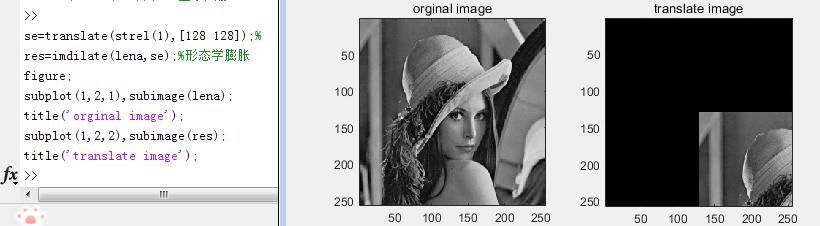figure;命令显示的变换后图片。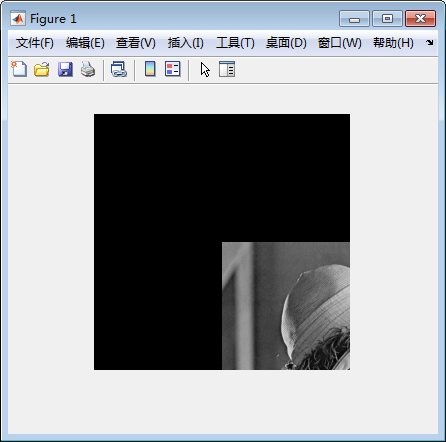https://blog.csdn.net/qwerasdf_1_2/article/details/54376657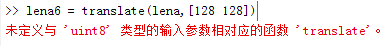strel(shape,parameters)。shape为形状参数，即设置什么样的结构元素；parameters为控制形状参数大小方向的参数。

SE =STREL('arbitrary',NHOOD)   创建一个指定邻域的平面结构化元素。NHOOD是一个包含1/0的矩阵；1的位置定义了邻域的形态学操作。NHOOD的中心就是它的中心元素，位置在FLOOR((SIZE(NHOOD) + 1)/2)。你也可以忽略参数串'arbitrary'而只使用STREL(NHOOD)。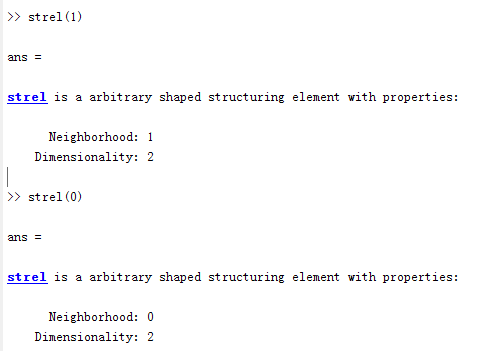随后实验证明strel（n）（n>1）都是和1一样的邻域大小

`J` = imdilate(`I`,`SE`)

`J = imdilate(I,nhood)`

`个人猜想：`

`以上思路就应当是想要使用translate 但是图像不是polyshape结构，所以先创造一个通用的结构元素（类似于java里的object），进行平移后再使用形态学函数填充结构元素。`

`在查阅上述原理的时候，由translate想到有没有支持通用图像的平移函数呢？毕竟是强大的matlab`

`果然找到了！`

`imtranslate`

`参考文档：`https://www.mathworks.com/help/images/ref/imtranslate.html

`一步到位版：`

``````lena6 = imtranslate(lena,[128, 128],'FillValues',255);%底色填充为白色
lena6 = imtranslate(lena,[128, 128],'FillValues',0);%底色填充为黑色

>> [R, C] = size(lena); % 获取图像大小
>> lena6 = imtranslate(lena,[R*0.5,C*0.5],'FillValues',0);
>> imshow(lena6);``````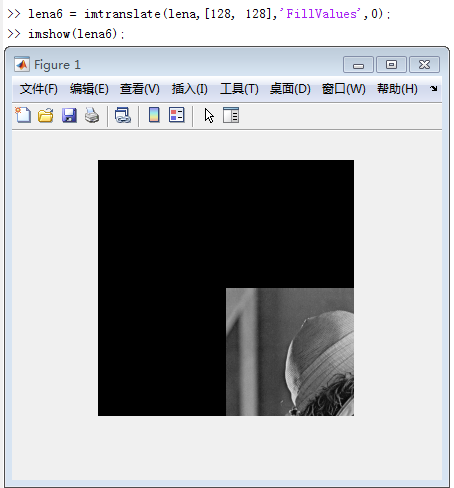matlab简单图像处理 相关内容